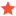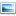• 0 Vote(s) - 0 Average
• 1
• 2
• 3
• 4
• 5
 Setting Minimum distance from objectAbdullahShafique27Junior MemberPosts: 3 Threads: 1 Joined: Feb 2023 Reputation: 0 02-19-2023, 06:05 PM I have been trying to figure out a way to set a minimum distance from my tool to a part as it moves through a program. As the parts that are used are complex and varying diffrent sizes of objects are used I want to automate this process. I assumed that RoboDK would have some sort of constraint built in for this but it seems not, so I have decided to try creating a py script. my code it below Code:```from robolink import * from robodk import * # Connect to RoboDK RDK = Robolink() # Get the robot and tool and object robot = RDK.Item('KUKA KR 6 R900-2') tool = RDK.Item('Kinect') object = RDK.Item('Piston deform') # Set the minimum distance constraint min_distance = 5000  # Set the desired minimum distance in millimeters while True:    # Get the pose of the robot, tool, and object    robot_pose = robot.Pose()    tool_pose = tool.PoseTool()    object_pose = object.Pose()    # Calculate the position of the tool in the global reference frame    tool_pose_global = robot_pose*tool_pose    # Calculate the distance between the tool and object    dist = distance(tool_pose_global.Pos(), object_pose.Pos())    # If the distance is less than the minimum distance, move the robot away from the object    if dist < min_distance:        displacement = min_distance - dist        direction = (tool_pose_global.Pos() - object_pose.Pos()) / distance        new_pos = robot_pose * transl(displacement * direction)        robot.MoveJ(new_pos)``` I seem to be having problems with the third last line of my code that starts direction as  I get an error as such: direction = (tool_pose_global.Pos() - object_pose.Pos()) / distance TypeError: unsupported operand type(s) for -: 'list' and 'list'  my simulation is trying to scan an object on a turntable with a camera from a  set distance from the surface of the complex object as it moves from a front facing orientation with respect to the object to an almost top down view with respect to the object as seen in the attached picture. I am not very good with programming in general and this is my first attempt in RoboDK. I am not sure if my code will even work if this error is fixed so I was wondering if anyone could help, or if there might be an easier way to do this.    Attached Files Thumbnail(s)Find ReplyAlbertModeratorPosts: 1,816 Threads: 1 Joined: Apr 2018 Reputation: 97 02-20-2023, 12:16 PM (This post was last modified: 02-20-2023, 12:16 PM by Albert.) You are using add/subract/multiply operators on a 3-dimensional list of values (3D vectors). You should use the required functions to perform these operations on 3D vectors. Example: Code:```delta_vector = subs3(tool_pose_global.Pos(), object_pose.Pos()) direction = mult3(delta_vector, 1.0/distance)```More information here: https://robodk.com/doc/en/PythonAPI/robo...math.subs3 Find ReplyAbdullahShafique27Junior MemberPosts: 3 Threads: 1 Joined: Feb 2023 Reputation: 0 02-21-2023, 10:18 AM (This post was last modified: 02-21-2023, 10:20 AM by AbdullahShafique27. Edit Reason: wronf code formatting ) I have changed my code slightly to incorpirate the new information you gave me as below Code:```from robolink import * from robodk import * # Connect to RoboDK RDK = Robolink() # Get the robot and tool and object robot = RDK.Item('KUKA KR 6 R900-2') tool = RDK.Item('Kinect') object = RDK.Item('Piston deform') # Set the minimum distance constraint min_distance = 5000  # Set the desired minimum distance in millimeters while True:    # Get the pose of the robot, tool, and object    robot_pose = robot.Pose()    tool_pose = tool.PoseTool()    object_pose = object.Pose()    # Calculate the position of the tool in the global reference frame    tool_pose_global = robot_pose*tool_pose    # Calculate the distance between the tool and object    dist = subs3(tool_pose_global.Pos(), object_pose.Pos())    newdist = mult3(dist,1.0)    # If the distance is less than the minimum distance, move the robot away from the object    if newdist < min_distance:        displacement = min_distance - newdist        direction = mult3(dist, 1.0/dist)        new_pos = robot_pose * transl(displacement * direction)        robot.MoveJ(new_pos)``` But now I am getting the error: Minimumdistance1.py", line 32, in                           if newdist TypeError: ' I am assuming this means it cant compare the dist < min_distance as they are stored as different types, but I am unsure how as I believe they are both scalar types from the code snippets below  Code:```newdist = mult3(dist,1.0) min_distance = 5000``` I think I am getting closer to a final script but the last few lines are causing me some problems. any advice would be great Find ReplyAlbertModeratorPosts: 1,816 Threads: 1 Joined: Apr 2018 Reputation: 97 02-22-2023, 09:46 AM You are using a vector as if it was the norm. You can calculate the norm of a vector by using the norm function: Code:`distance = norm(vector)` In your example: Code:`dist_norm = norm(dist)` Find ReplyAbdullahShafique27Junior MemberPosts: 3 Threads: 1 Joined: Feb 2023 Reputation: 0 02-22-2023, 04:22 PM I have further modified my code and now there are no errors when doing the quick program check. However when I set the minimum distance to larger than 760 I get a weird response as seen in the video attached. I am sure this has to do with the fact that my TVP is offset from the flange by 760mm, and the targets for the movement are based around the TCP changing angles around the parts reference frame which will change if the minimum distance is larger than the initial offset of 760mm.  I am unsure of if this is a problem with my code or I am going to have to use another way other than targets to define my path as it will change depending on the minimum distance I set and the scanning program I have made. My code is below if there are any obvious problems with it but I cant seem to see any. Code:```from robolink import * from robodk import * # Connect to RoboDK RDK = Robolink() # Get the robot and tool and object robot = RDK.Item('KUKA KR 6 R900-2') tool = RDK.Item('Kinect') object = RDK.Item('Piston deform') # Set the minimum distance constraint min_distance = 800  # Set the desired minimum distance in millimeters while True:    # Get the pose of the robot, tool, and object    robot_pose = robot.Pose()    tool_pose = tool.PoseTool()    object_pose = object.Pose()    # Calculate the position of the tool in the global reference frame    tool_pose_global = robot_pose*tool_pose    # Calculate the distance between the tool and object    dist = subs3(tool_pose_global.Pos(), object_pose.Pos())    newdist = norm(dist)    # If the distance is less than the minimum distance, move the robot away from the object    if newdist < min_distance:        displacement = min_distance - newdist        displacement = int(displacement)        direction = mult3(dist, 1.0/newdist)        new_pos = robot_pose * transl(displacement * direction)        robot.MoveJ(new_pos)``` Attached FilesRoboDK - 3d scanning - Free (Trial) - 23 days left 2023-02-22 16-09-43.mp4 (Size: 10.5 MB / Downloads: 10) Find Reply

 Users browsing this thread: 1 Guest(s)